# This document

The purpose of this vignette is to introduce readers to hclust1d package, and bring them up to speed by providing a simple use case example.

# Clustering in 1D

The name of hclust1d package stands for Hierarchical CLUSTering for 1D. 1D means that data is univariate or one dimensional, i.e. constitutes of real numbers. The package contains a suit of algorithms for univariate agglomerative hierarchical clustering. Clusters or clustering process in 1D is also called segmentation or breaks [Fisher, 1958 and Jenks, 1977] and arises naturally in research related to choropleth maps.

# Hierarchical clustering in a nutshell

Agglomerative hierarchical clustering first assigns each observation (1D point in our case) to a singleton cluster. Thus, we start off with as many clusters as there are observations. Then, in each step of the algorithm, the closest two clusters get merged. In order to decide, which clusters are closest, we need a way to measure either a distance, a dissimilarity or a similarity between clusters.

Below, for clarity, we will drop saying a distance, or a dissimilarity, or a similarity and will refer to a distance in this broader colloquial sense, in which for instance a triangle inequality may not hold.

Please note, that we start off with a measure of distance, but it works for observations only. So we need to generalize this initial measure to work for clusters, too. It is easy for singleton clusters - we simply say, that a distance (or a dissimilarity, or a similarity) between two singleton clusters is the same as between the two observations involved.

But in order to say what is a distance between more complex clusters, we need a concept of a linkage function. This concept constitutes a link between the distance for clusters and the distance between observations, hence its name.

For instance, we could say that a distance between two clusters $$A$$ and $$B$$ is the same as the minimal distance between any observation $$a \in A$$ and any observation $$b \in B$$. This would be called a single linkage in hierarchical clustering terminology.

Sometimes, instead of defining a cluster-wise distance in terms of distances between the clustered observations, it would be easier to build a distance concept for any two clusters (that are present in a current step of our hierarchical clustering procedure) inductively upon the distance concept as it got defined for smaller clusters. Observe, that we have it defined for singleton clusters already. Then, we could say, for instance, that after $$A$$ and $$B$$ got merged (denoted $$A \cup B$$) the distance between $$A \cup B$$ and any other cluster $$C$$ is the arithmetic average between two distances: the one between $$A$$ and $$C$$ and the one between $$B$$ and $$C$$. This would be called a mcquitty or WPGMA linkage clustering.

### Merging height

Now, that we understand a concept of a linkage function, the concept of closest clusters becomes clear, too. After the closest clusters get merged, the height of this merging is defined as their cluster-wise distance. Obviously not only the choice of the closest clusters, but also the merging height, they both depend on a choice of a linkage function.

hclust1d supports a comprehensive list of choices of a linkage function, matching all possible choices in stats::hclust with an addition of a true_median linkage.

Below find a complete list of all linkage functions supported by hclust1d. We also state what is a distance of the newly merged cluster $$A \cup B$$ and some other cluster $$C$$. Sometimes it can be done in terms of the observations involved, and sometimes an inductive definition is easier. Below, the distance function is denoted $$d(\cdot, \cdot)$$ and it works for observations, and with a slight abuse of notation for clusters or for arbitrary points; the number of observations in a cluster $$X$$ is denoted $$\left | X \right |$$.

• complete: a distance between two clusters is the maximum distance between all pairs of observations in the involved clusters, formally $$d(A \cup B, C) = \max_{x \in A \cup B,\,y \in C}d(x,y)$$.

• single: a distance between two clusters is the minimum distance between all pairs of observations in the involved clusters, formally $$d(A \cup B, C) = \min_{x \in A \cup B,\,y \in C}d(x,y)$$.

• average, called also UPGMA (Unweighted Pair Group Method with Arithmetic mean): a distance between two clusters is the (arithmetic) average distance between all pairs of observations in the involved clusters, formally $$d(A \cup B, C) = \frac{\sum_{x \in A \cup B} \sum_{y \in C} d(x,y)}{\left | A \cup B \right | \cdot \left | C \right |}$$.

• centroid, called also UPGMC (Unweighted Pair Group Method with Centroid average): $$d(A \cup B, C) = \left \| \frac{ \sum_{x \in A \cup B} x }{\left | A \cup B \right |} - \frac{ \sum_{y \in C} y }{\left | C \right |} \right \| ^2$$. Observe, that centroid linkage reports height as the squared euclidean distance between clusters’ centroids. With $$d(\cdot, \cdot)$$ being an euclidean distance, this can be rewritten as $$d(A \cup B, C) = d \left( \frac{ \sum_{x \in A \cup B} x}{\left | A \cup B \right |}, \frac{ \sum_{y \in C} y}{\left | C \right |} \right ) ^2$$.

• median, called also WPGMC (Weighted Pair Group Method with Centroid average): $$d(A \cup B, C) = d(m_{A \cup B}, m_C)^2$$ with $$m_X$$ equal to the observation in cases of $$X$$ being a singleton, and $$m_{A \cup B} = \frac{1}{2}\left (m_A + m_B \right )$$ for merged clusters $$A$$ and $$B$$. Observe, that median linkage reports height as the squared distance, similarly to centroid linkage. Observe also, that this definition is in odds with the Wikipedia hierarchical clustering page, and although it is compatible with stats::hclust, this behavior is not well documented in stats::hclust, either.

• mcquitty, called also WPGMA (Weighted Pair Group Method with Arithmetic mean): $$d(A \cup B, C) = \frac{d(A, C) + d(B, C)}{2}$$

• ward.D, called also MISSQ (Minimum Increase of Sum of SQuares): $$d(A \cup B, C) = 2 \cdot \frac{\left | A \cup B \right | \cdot \left | C \right |}{\left | A \cup B \cup C \right |} \cdot \left \| \frac{ \sum_{x \in A \cup B} x}{\left | A \cup B \right |} - \frac{ \sum_{y \in C} y}{\left | C \right |} \right \| ^2$$. Observe, that ward.D linkage reports height as the squared euclidean distance between clusters’ centroids weighted with a harmonic mean of relevant clusters’ sizes. This definition is in odds with what one can read in the Wikipedia hierarchical clustering page. With $$d(\cdot, \cdot)$$ being an euclidean distance, this can be rewritten as $$d(A \cup B, C) = 2 \cdot \frac{\left | A \cup B \right | \cdot \left | C \right |}{\left | A \cup B \cup C \right |} \cdot d \left( \frac{ \sum_{x \in A \cup B} x}{\left | A \cup B \right |}, \frac{ \sum_{y \in C} y}{\left | C \right |} \right ) ^2$$.

• ward.D2: added to stats::hclust in R >3.0.3 versions to implement the original Ward’s linkage function [Murtagh and Legendre, 2014] which is not implemented with ward.D. The reported height in ward.D2 is the square root of the height in ward.D, i.e. $$d(A \cup B, C) = \sqrt{ 2 \cdot \frac{\left | A \cup B \right | \cdot \left | C \right |}{\left | A \cup B \cup C \right |} } \cdot \left \| \frac{ \sum_{x \in A \cup B} x}{\left | A \cup B \right |} - \frac{ \sum_{y \in C} y}{\left | C \right |} \right \|$$. So ward.D2 linkage reports height as the unsquared euclidean distance between clusters’ centroids weighted with a square root of harmonic mean of relevant clusters’ sizes. With $$d(\cdot, \cdot)$$ being an euclidean distance, this can be rewritten as $$d(A \cup B, C) = \sqrt{2 \cdot \frac{\left | A \cup B \right | \cdot \left | C \right |}{\left | A \cup B \cup C \right |} } \cdot d \left( \frac{ \sum_{x \in A \cup B} x }{\left | A \cup B \right |}, \frac{ \sum_{y \in C} y }{\left | C \right |} \right )$$.

• true_median: $$d(A \cup B, C) = d(m_{A \cup B}, m_C)$$ with $$m_X$$ being the median of observations in $$X$$ (specifically, the middle-valued observation in case of $$\left | X \right |$$ odd, and the arithmetic mean of the two middle-valued observations in case of $$\left | X \right |$$ even). Note also, that a concept of a median makes sense only for 1D points.

### Computational complexity of 1D hierarchical clustering

Hierarchical clustering in the 1D setting has time complexity of $$\mathcal{O}(n\log n)$$ time regardless of the linkage function used.

Compatibility with stats::hclust was high in the priority list and thus for 1D data it is simply a matter of a plug-and-play replacement of stats::hclust calls to be able to use the advantage of our fast implementation of this asymptotically much more efficient algorithm. The how-to is covered in detail in our replacing stats::hclust vignette.

# OK, so let’s get started

To load the package into memory execute the following line:

library(hclust1d)

We will work with random normally distributed data points in this vignette.

points <- rnorm(10)

Working with hclust1d is very simple an requires only passing the data points vector and optionally a linkage method to hclust1d function (complete linkage is used as a default, if the linkage method is not provided). The simplest example of a complete linkage clustering:

result <- hclust1d(points)

The hclust1d function returns an object of the same type that is returned from stats::hclust (a list object with S3 class , to be specific).

• This makes it straightforward to further work with the clustering results. E.g. we can plot it (observe that plot.hclust gets called internally below):

plot(result)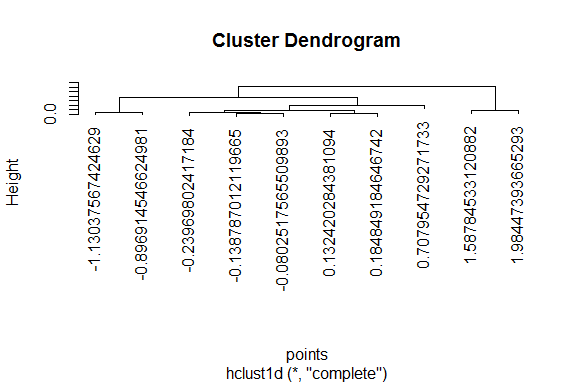• We can also generate clustering for the named 1D points:

names(points) <- paste("point", 0:9, sep = "-")
result <- hclust1d(points)
plot(result)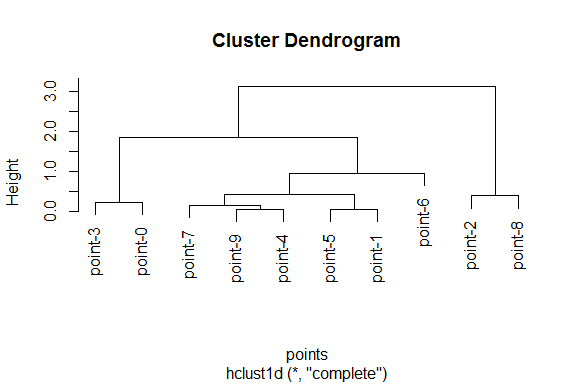• We can print the clustering result:

print(result)
#>
#> Call:
#> hclust1d(x = points)
#>
#> Cluster method   : complete
#> Distance         : euclidean
#> Number of objects: 10
• Or we can convert the clustering result to a dendrogram and further work with it (observe that plot.dendrogram gets called internally below):

dendrogram <- as.dendrogram(result)
plot(dendrogram, type = "triangle", ylab = "Height")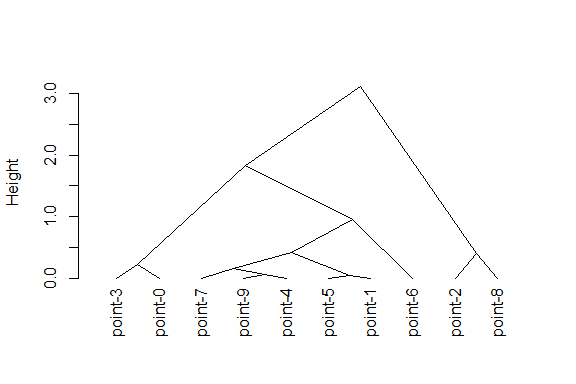• Or we can use any other specialized packages, like ggdendro with ggplot2 or ape packages, to further visualize and work with the result.

# How to change the linkage function used for clustering

By default, complete linkage is used for clustering. But it is very straightforward to explicitly say which linkage function is to be used. You just need to specify a method argument of the hclust1d call, as in example below with mcquitty linkage function. Observe that the linkage function name is passed as a character string:

result <- hclust1d(points, method = "mcquitty")
plot(result)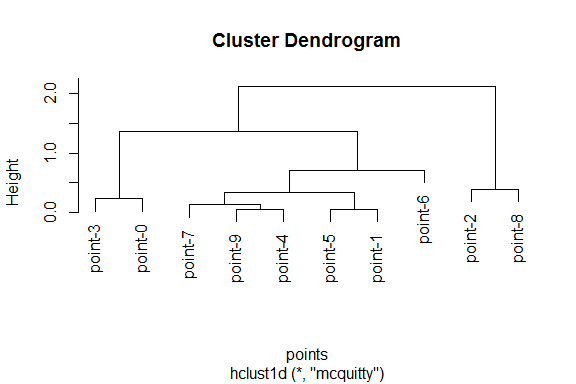# Should I provide points or rather a distance matrix to hclust1d?

In a default statistical package in R, stats::hclust requires that the dist structure is provided for clustering. In fact, stats::hclust cannot be executed on raw $$\mathbb{R}^d$$ points.

However, hclust1d is more flexible in this regard. It accepts both $$\mathbb{R}^d$$ points as input (with $$d=1$$, because hclust1d works only in 1D setting) and a dist S3 class input. Actually, the raw points are recreated from a distance structure anyway, so raw point input is preferred and works a little bit faster.

If you want to provide the dist structure, please remember to change a distance argument to TRUE in a call to hclust1d. The two examples below return results that are equivalent (but not equal - a note on that follows below).

### Examples

For diversity, single linkage is used in the two examples below:

result_points <- hclust1d(points, method = "single")
plot(result_points)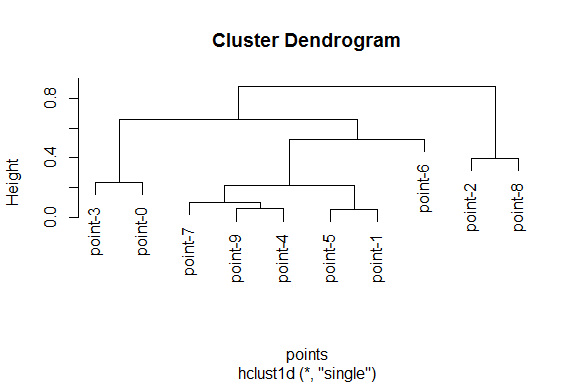distances <- dist(points)

result_dist <- hclust1d(distances, distance = TRUE, method = "single")
plot(result_dist)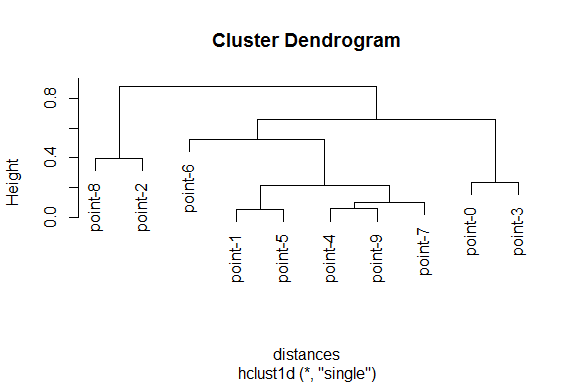But are the results the same? On a close inspection, you’ll notice that the resulting clusterings are mirror reflections of each other. It is perfectly OK, the distance structure specifies the mutual distances, but not the order or shift of points, so the resulting clusterings are equivalent up to the order and shift.

In a default statistical package in R, stats::hclust requires that the squared dist structure is provided for ward.D, centroid and median linkage functions. It is also reflected in merging height resulting in those linkages: the height is returned as the appropriate distance measure, squared.

Again, hclust1d is more flexible in this regard. It accepts both squared and unsquared distances (squared or unsquared dist S3 class input), and it even accepts raw $$\mathbb{R}^d$$ points as input (with $$d=1$$, because hclust1d works only in 1D setting). Actually, the raw points are preferred in this setting, too.

The below points should be observed:

• If you want to provide the unsquared dist structure, please remember to change a distance argument to TRUE in a call to hclust1d.
• If you want to provide the squared dist structure, please remember to change both distance and squared arguments to TRUE in a call to hclust1d.

### Examples

result_points <- hclust1d(points, method = "ward.D")
plot(result_points)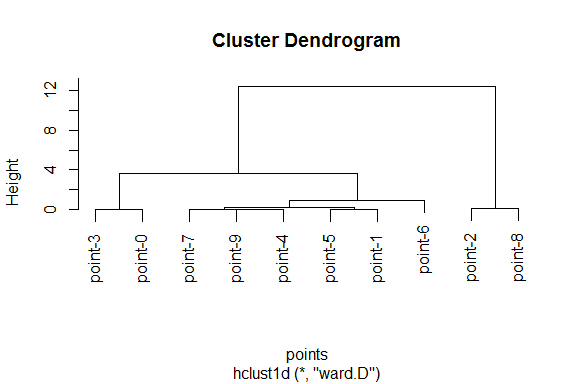distances <- dist(points)

result_dist <- hclust1d(distances, distance = TRUE, method = "ward.D")
plot(result_dist)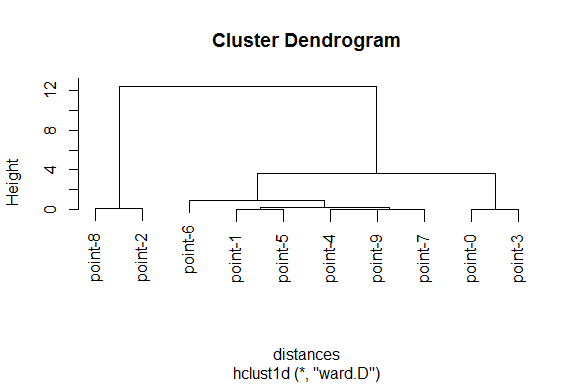squared_distances <- distances ^ 2

result_dist <- hclust1d(squared_distances, distance = TRUE, squared = TRUE, method = "ward.D")
plot(result_dist)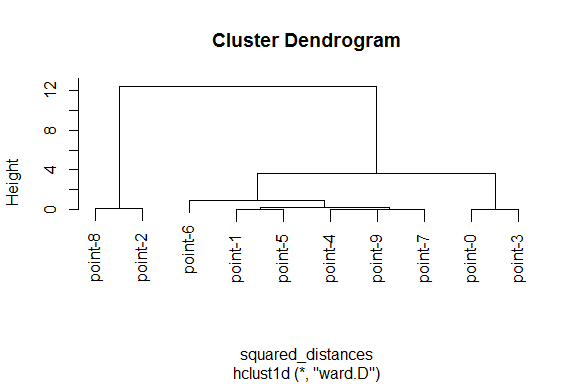The three examples above return results that are equivalent (up to the order and shift, because again you will notice, that the first clustering is a mirror reflection of the second and the third clusterings).

Please also note, that regardless of input, the height is returned as the appropriately squared distance measure for ward.D, centroid and median linkage functions, so the results remain compatible with stats::hclust results for the squared dist input (for those linkages). Please check the result below, for comparison. It presents the same clustering with the same heights, only the presented order of points is reorganized.

result_dist_stats_hclust <- stats::hclust(squared_distances, method = "ward.D")
plot(result_dist_stats_hclust)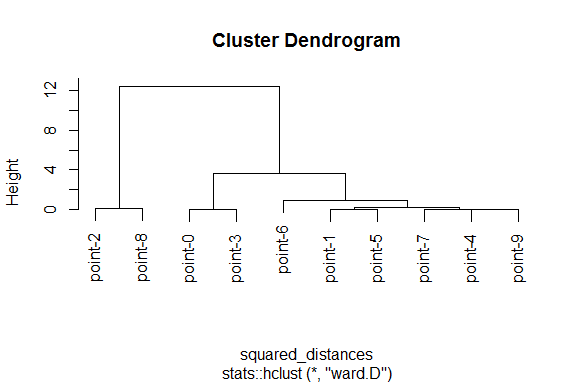### A note on ward.D2 and ward.D

There is a lot of confusion on ward.D and ward.D2 linkages on Internet. Maybe not so surprisingly, the difference is very simple: the returned merging heights in ward.D are squared, while in ward.D2 they are not. You can see it by comparing the following output:

distances <- dist(points)

result_ward.D <- hclust1d(distances, distance = TRUE, method = "ward.D")
result_ward.D2 <- hclust1d(distances, distance = TRUE, method = "ward.D2")

print(result_ward.D$height) #>  0.002748790 0.003426376 0.022595304 0.054504098 0.157314251 #>  0.232947678 0.903435672 3.683388750 12.403250822 print(result_ward.D2$height ^ 2)
#>   0.002748790  0.003426376  0.022595304  0.054504098  0.157314251
#>   0.232947678  0.903435672  3.683388750 12.403250822

Unfortunately, stats::hclust adds another layer of confusion, by requiring implicitly that the input is provided as squared distances for ward.D and unsquared for ward.D2. Let’s try to recreate the above outputs by using stats::hclust:

distances <- dist(points)
squared_distances <- distances ^ 2

result_ward.D_stats_hclust <- stats::hclust(squared_distances, method = "ward.D")
result_ward.D2_stats_hclust <- stats::hclust(distances, method = "ward.D2")

print(result_ward.D_stats_hclust$height) #>  0.002748790 0.003426376 0.022595304 0.054504098 0.157314251 #>  0.232947678 0.903435672 3.683388750 12.403250822 print(result_ward.D2_stats_hclust$height ^ 2)
#>   0.002748790  0.003426376  0.022595304  0.054504098  0.157314251
#>   0.232947678  0.903435672  3.683388750 12.403250822

The distinction that stats::hclust makes about its input (squared or unsquared) is unfortunately implicit, not explicit.

# Cutting heights

The last topic presented in this introductory vignette is how to get an actual single clustering from the dendrogram. You’ll notice, that a dendrogram in hierarchical clustering contains multiple clusterings: there is a clustering into one cluster only, there is a clustering into two clusters and ultimately, there is a clustering into as many clusters as there are observations. The number of actual clusters in a clustering depends on a height that a dendrogram tree is cut.

We will use a standard stats::cutree function to cut the results of hclust1d, because those results are fully compatible with the results of stats::hclust. One can cut a dendrogram either at a given height, or specifying a desired number of clusters.

But before we get into cutting, let’s first examine a complete linkage clustering full dendrogram:

result <- hclust1d(points)
plot(result)The exact merging height values may not be apparent from the dendrogram plot. They are as follows:

print(result$height) #>  0.05242890 0.05853526 0.15944627 0.23346113 0.39662861 0.42454721 0.94765275 #>  1.83833040 3.11484961 The closest points are point-1 and point-5 with a distance of 0.0524289 and thus merged at the first height (valued 0.0524289). So if you cut the dendrogram at 0.0524289 you’ll get $$n-1$$ clusters, with $$n$$ equal to the number of points, $$n=10$$ in this example. n_minus_one_clusters <- stats::cutree(result, h = result$height)
print(n_minus_one_clusters)
#> point-0 point-1 point-2 point-3 point-4 point-5 point-6 point-7 point-8 point-9
#>       1       2       3       4       5       2       6       7       8       9

In this example you can see how the clusters get assigned: each point in a vector gets assigned a cluster number, with both point-1 and point-5 being assigned to the same cluster number 2, and all other points having their own singleton cluster.

There are $$n-1$$ merging heights for $$n$$ points, in our case there are 9 heights. At the last 9-th height, valued 3.1148496, the last two clusters get merged and we have only one cluster at that height:

one_cluster <- stats::cutree(result, h = result\$height)
print(one_cluster)
#> point-0 point-1 point-2 point-3 point-4 point-5 point-6 point-7 point-8 point-9
#>       1       1       1       1       1       1       1       1       1       1

Let’s say our goal is to get 3 clusters. We should cut the dendrogram at any height between the 7-th height 0.9476528 (inclusive) and the 8-th height 1.8383304 (exclusive). Let’s cut the dendrogram at the height 1.0:

three_clusters <- stats::cutree(result, h = 1.0)
print(three_clusters)
#> point-0 point-1 point-2 point-3 point-4 point-5 point-6 point-7 point-8 point-9
#>       1       2       3       1       2       2       2       2       3       2

Alternatively, one can cut the dendrogram specifying not the cutting height, but rather explicitly the desired number of clusters. You can specify the desired number of clusters with a k argument to cutree:

alt_three_clusters <- stats::cutree(result, k = 3)
print(alt_three_clusters)
#> point-0 point-1 point-2 point-3 point-4 point-5 point-6 point-7 point-8 point-9
#>       1       2       3       1       2       2       2       2       3       2

How to read the clustering resulting from this cut? As we said earlier, each point gets assigned a cluster number, so in this last example we get the following clustering (into three clusters):

• the first cluster with 2 points: point-0 and point-3,

• the second cluster with 6 points: point-1, point-4, point-5, point-6, point-7 and point-9,

• the third cluster with 2 points: point-2 and point-8.

Have a look at the dendrogram plot above to verify that indeed a cut at the height 1.0, or (equivalently) into 3 clusters, would result in this clustering structure.